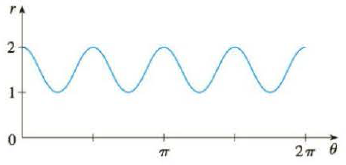Chapter 10.3, Problem 48E

Chapter
Section
Textbook Problem

# The figure shows a graph of r as a function of θ in Cartesian coordinates. Use it to sketch the corresponding polar curve.48.To determine

To sketch: Polar curve from the given Cartesian curve shown below in figure 1.

Explanation

Given:

The polar curve is shown below in figure 1.

Calculation:

From the figure 1, when θ increases from 0 to π4 , polar curve r decreases from 2 to 1 in polar graph.

When θ increases from π4 to π2 , polar curve r increases from 1 to 2 .

When θ increases from π2 to 3π4 , polar curve r decreases from 2 to 1 .

When θ increases from 3π4 to π , polar curve r increases from 1 to 2 .

When θ increases from π to 5π4 , polar curve r decreases from 2 to 1

### Still sussing out bartleby?

Check out a sample textbook solution.

See a sample solution

#### The Solution to Your Study Problems

Bartleby provides explanations to thousands of textbook problems written by our experts, many with advanced degrees!

Get Started

#### Find more solutions based on key concepts